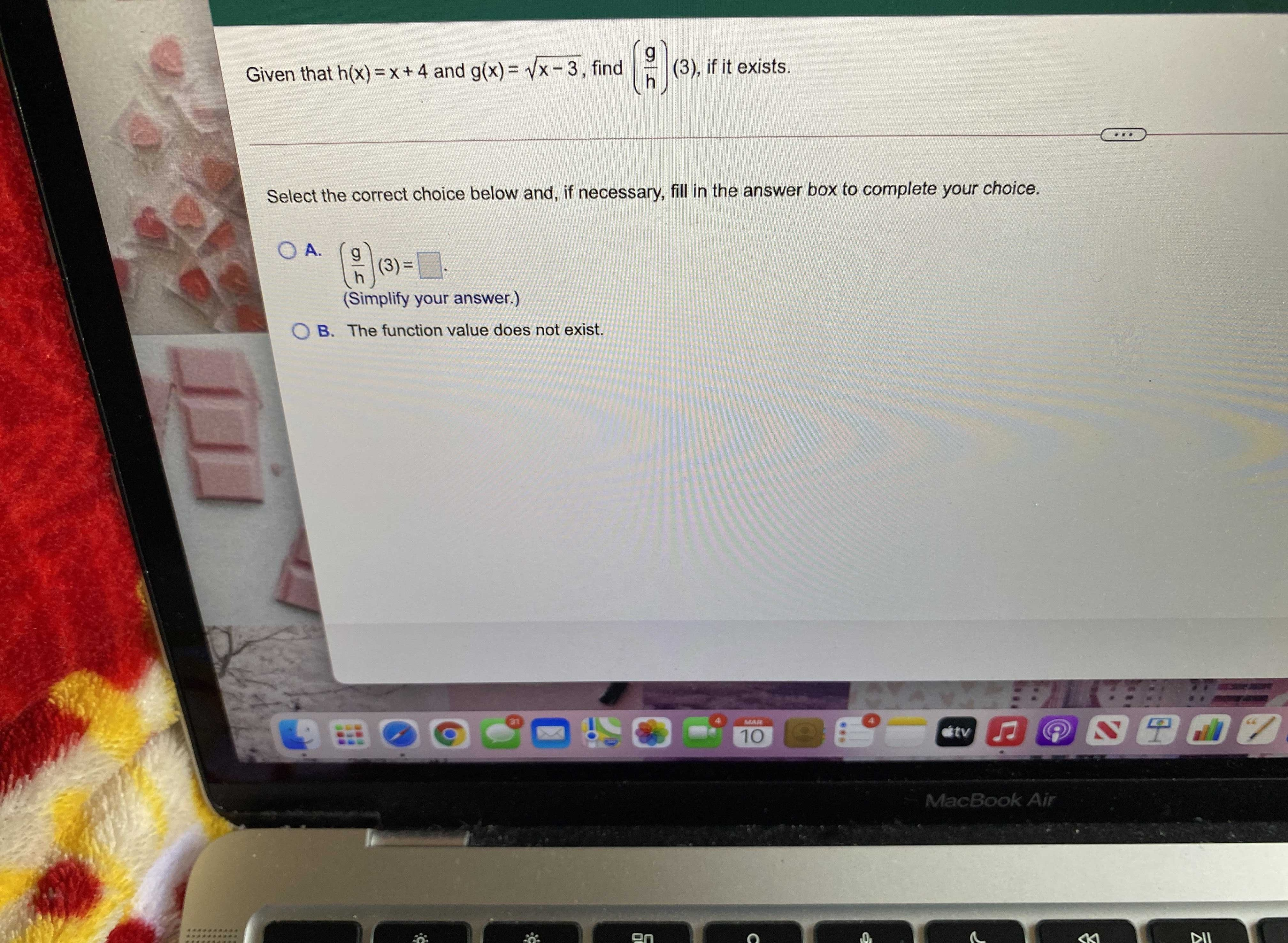### Still have math questions?

Algebra
QuestionGiven that $$h ( x ) = x + 4$$ and $$g ( x ) = \sqrt { x - 3 } ,$$ find $$( \frac { g } { h } ) ( 3 )$$ , if it exists. Select the correct choice below and, if necessary, fill in the answer box to complete your choice. A. $$( \frac { g } { h } ) ( 3 ) =$$

$$(\frac{g}{h} )(3)= 0$$